## 串联和并联电阻器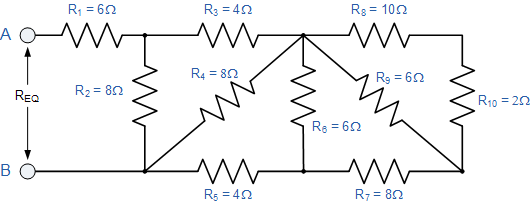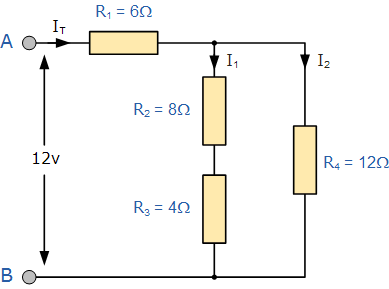R 2  + R 3  =8Ω+4Ω=12Ω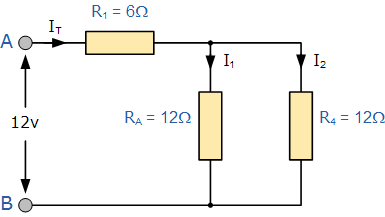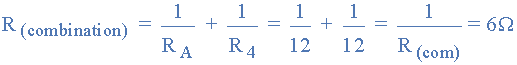[R= R comb  + R 1  =6Ω+6Ω=12Ω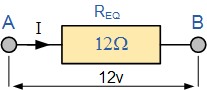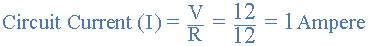V （R1） = I * R 1 = 1 * 6 = 6伏

V （RA） = V R4 =（12-V R1）= 6伏

I 1 = 6V÷R A = 6÷12 = 0.5A或500mA

I 2 = 6V÷R 4 = 6÷12 = 0.5A或500mA